## Analogy

#### Analogy

Direction: Each of the following questions consists of two sets of figures. Figures (a), (b), (c) and (d) constitute the Question Set while figures (1), (2), (3), (4) and (5) constitute the Answer Set. There is a definite relationship between figures (a) and (b). Figure (a) and (b) are related in a particular way or manner. Establish the same relationship between (c) and (d).

1. Select a suitable figure from the answer figures that would replace the question mark (?) from question figure.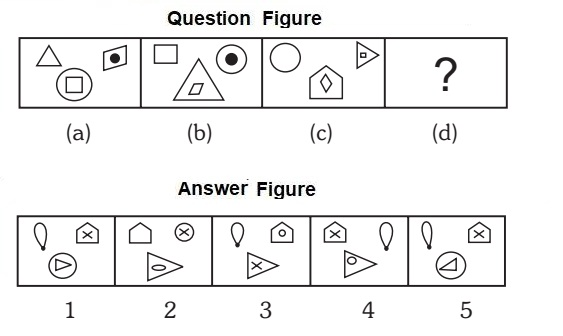1. The upper left element becomes the lower outer element, the outer part of the upper right element becomes the lower –inner element and the symbol inside the upper right element remains in its place, the lower –inner element becomes the upper left element, the lower outer element becomes the outer part of the upper –right element.

##### Correct Option: A

The upper left element becomes the lower outer element, the outer part of the upper right element becomes the lower –inner element and the symbol inside the upper right element remains in its place, the lower –inner element becomes the upper left element, the lower outer element becomes the outer part of the upper –right element.

1. Select a suitable figure from the answer figures that would replace the question mark (?) from question figure.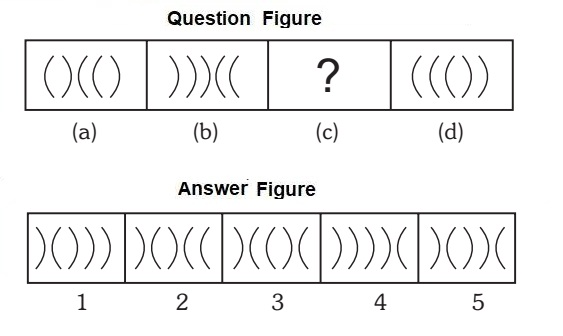1. The first, second and fifth arcs (counting from left to right) get laterally inverted.

##### Correct Option: E

The first, second and fifth arcs (counting from left to right) get laterally inverted.

1. Select a suitable figure from the answer figures that would replace the question mark (?) from question figure.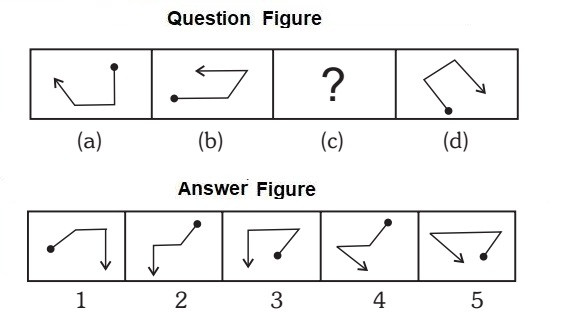1. The pin rotates 90 degree clock wise, the arrow rotates 45 degree clock wise and the line joining the pin and the arrow rotates 135 degree.

##### Correct Option: C

The pin rotates 90 degree clock wise, the arrow rotates 45 degree clock wise and the line joining the pin and the arrow rotates 135 degree.

1. Select a suitable figure from the answer figures that would replace the question mark (?) from question figure.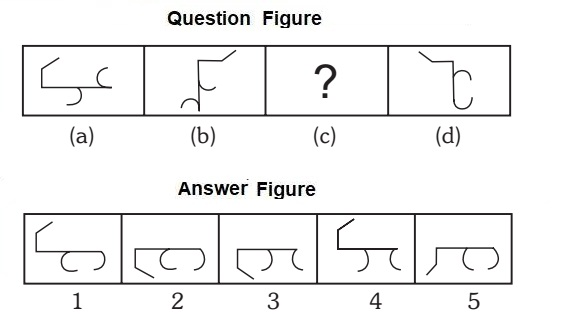##### Correct Option: B

1. Identify the two figures which are similar in all respects.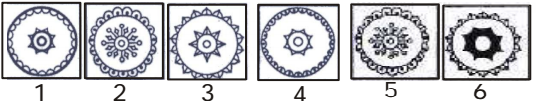1. Figure (2)and (5) are similar in all respects.

##### Correct Option: D

Figure (2)and (5) are similar in all respects.